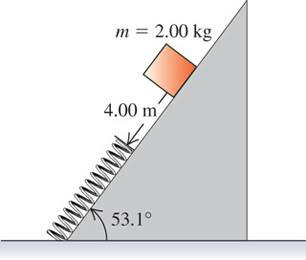# Elastic force on an incline

• cycam

## Homework Statement

A 2.00-kg package is released on a 53.1 ^\circ incline, 4.00 m from a long spring with force constant 120 N/m that is attached at the bottom of the incline View Figure . The coefficients of friction between the package and the incline are \mu_{s} \;=\; 0.40 and \mu_{k} \;=\; 0.20. The mass of the spring is negligible.1.] What is the speed of the package just before it reaches the spring?

2.] What is the maximum compression of the spring?

3.] The package rebounds back up the incline. How close does it get to its initial position?

## The Attempt at a Solution

I only have a limited amount of attempts to input the correct solution and I already have tried a couple different ways. I want to be guided in the right direction, not have the answer handed to me, please. Any help would be greatly appreciated. Thank youThe forces that act on the block are friction, gravity. You can calculate the force along the incline and use the kinematics equations to find the velocity (if you have the force, you'll have the acceleration).

For the compression of the spring, you have the velocity, and hence the kinetic energy. That converts into the potential energy of the spring AND the work done against friction.

For the last part, again use the same considerations to find the max height of the block.

Btw, sin53=4/5 and cos53=3/5

argh. i can't seem to get the second part.
my equation is...
1/2kx^2 + (u_k)mgcos(53.1) - (1/2)(m)(v^2)=0
can anyone help?

Yeah,

The equation works out to be:
$$0.5kx^2=4mgsin(53) - \mu _k mg*cos53*4$$